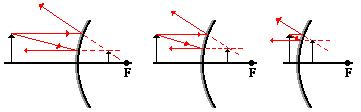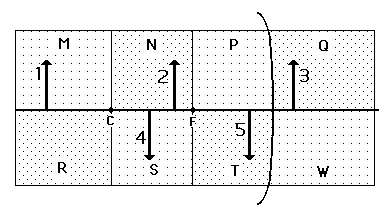Reflection and the Ray Model of Light - Lesson 4 - Convex Mirrors

# Image Characteristics for Convex Mirrors

Previously in Lesson 4, ray diagrams were constructed in order to determine the location, size, orientation, and type of image formed by concave mirrors. The ray diagram constructed earlier for a convex mirror revealed that the image of the object was virtual, upright, reduced in size and located behind the mirror. But will these always be the characteristics of an image produced by a convex mirror? Can convex mirrors ever produce real images? Inverted images? Magnified Images? To answer these questions, we will look at three different ray diagrams for objects positioned at different locations along the principal axis. The diagrams are shown below.The diagrams above show that in each case, the image is

• located behind the convex mirror
• a virtual image
• an upright image
• reduced in size (i.e., smaller than the object)

Unlike concave mirrors, convex mirrors always produce images that share these characteristics. The location of the object does not affect the characteristics of the image. As such, the characteristics of the images formed by convex mirrors are easily predictable.

Another characteristic of the images of objects formed by convex mirrors pertains to how a variation in object distance affects the image distance and size. The diagram below shows seven different object locations (drawn and labeled in red) and their corresponding image locations (drawn and labeled in blue).The diagram shows that as the object distance is decreased, the image distance is decreased and the image size is increased. So as an object approaches the mirror, its virtual image on the opposite side of the mirror approaches the mirror as well; and at the same time, the image is becoming larger.

### We Would Like to Suggest ...Why just read about it and when you could be interacting with it? Interact - that's exactly what you do when you use one of The Physics Classroom's Interactives. We would like to suggest that you combine the reading of this page with the use of our Optics Bench Interactive or our Name That Image Interactive. You can find this in the Physics Interactives section of our website. The Optics Bench Interactive provides the learner an interactive enivronment for exploring the formation of images by lenses and mirrors. The Name That Image Interactive provides learners with an intensive mental workout in recognizing the image characteristics for any given object location in front of a curved mirror.

The following questions pertain to the image characteristics of all types of mirrors discussed in this unit - plane mirrors, concave mirrors, and convex mirrors. Use your understanding of the object-image relationships for these three types of mirrors to answer the questions.

The diagram below shows a spherical surface that is silvered on both sides. Thus, the surface serves as double-sided mirror, with one of the sides being the concave and one being the convex side. The principal axis, focal point, and center of curvature are shown. The region on both sides of the mirror is divided into eight sections (labeled M, N, P, Q, R, S, T, and W). Five objects (labeled 1, 2, 3, 4, and 5) are shown at various locations about the double-sided mirror. Use the diagram to answer the questions #1-6.1. The image of object 1 would be located in section ______.

 M N P Q R S T W

2. The image of object 2 would be located in section ______.

 M N P Q R S T W

3. The image of object 3 would be located in section ______.

 M N P Q R S T W

4. The image of object 4 would be located in section ______.

 M N P Q R S T W

5. The image of object 5 would be located in section ______.

 M N P Q R S T W

6. The double-sided mirror would cause virtual image to be formed of objects ________.

 a. 1, 2, and 4 b. 1, 2, and 3 c. 3 and 5 d. 4 and 5 e. 3 only7. How can a plane mirror, concave mirror, and/or convex mirror be used to produce an image that has the same size as the object?

8. How can a plane mirror, concave mirror, and/or convex mirror be used to produce an upright image?

9. How can a plane mirror, concave mirror, and/or convex mirror be used to produce a real image?

10. The image of an object is found to be upright and reduced in size. What type of mirror is used to produce such an image?

Next Section: